13 de Mar de 2023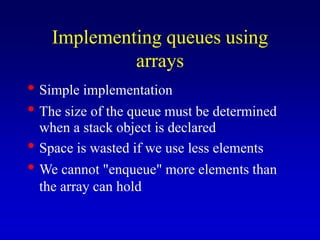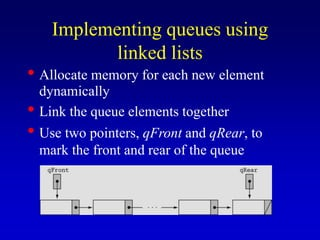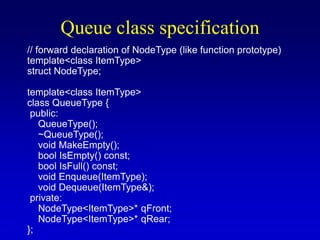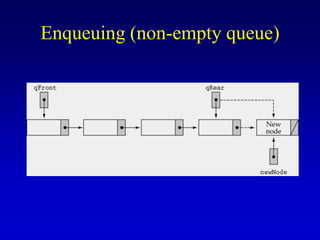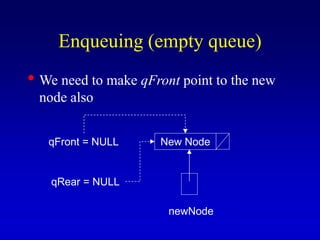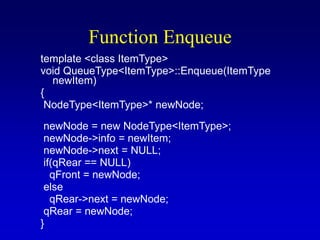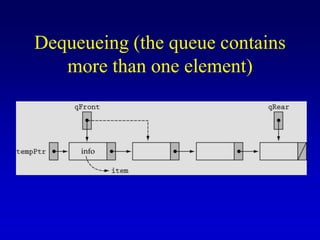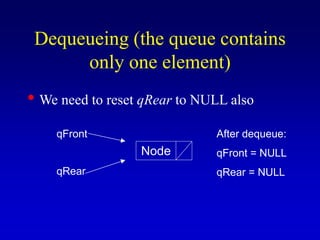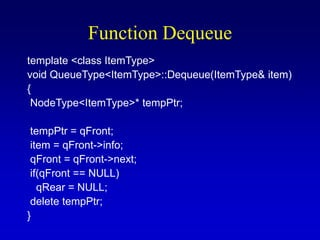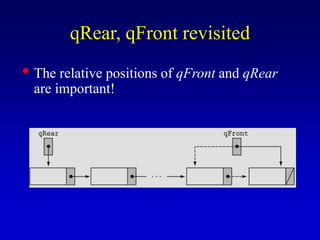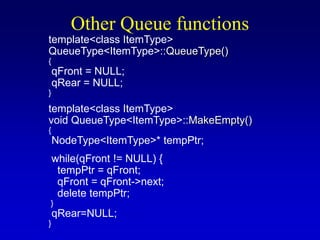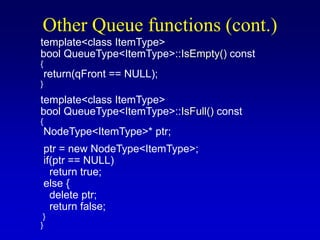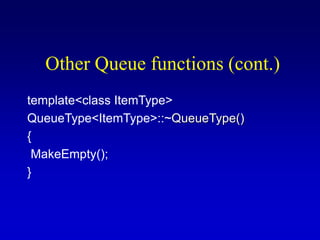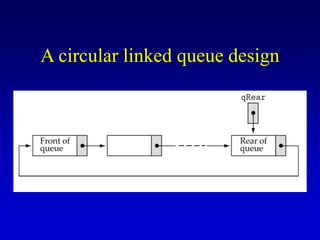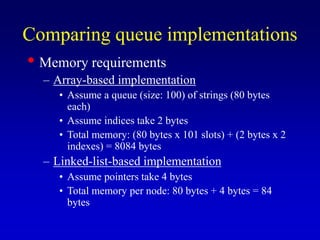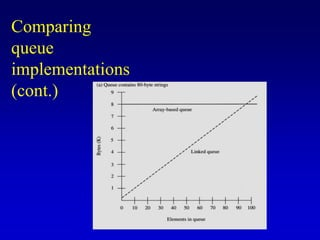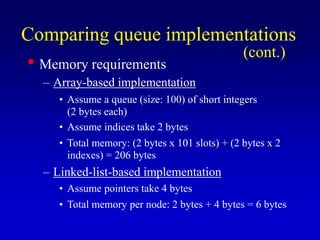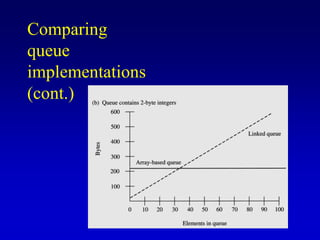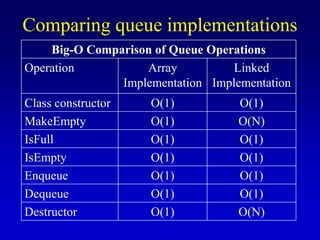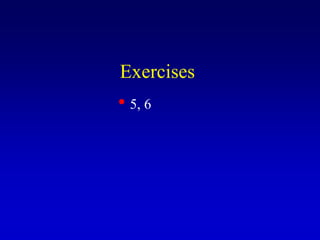1 de 21

• 1. Implementing a Queue as a Linked Structure CS 308 – Data Structures
• 2. Implementing queues using arrays • Simple implementation • The size of the queue must be determined when a stack object is declared • Space is wasted if we use less elements • We cannot "enqueue" more elements than the array can hold
• 3. Implementing queues using linked lists • Allocate memory for each new element dynamically • Link the queue elements together • Use two pointers, qFront and qRear, to mark the front and rear of the queue
• 4. Queue class specification // forward declaration of NodeType (like function prototype) template<class ItemType> struct NodeType; template<class ItemType> class QueueType { public: QueueType(); ~QueueType(); void MakeEmpty(); bool IsEmpty() const; bool IsFull() const; void Enqueue(ItemType); void Dequeue(ItemType&); private: NodeType<ItemType>* qFront; NodeType<ItemType>* qRear; };
• 5. Enqueuing (non-empty queue)
• 6. Enqueuing (empty queue) • We need to make qFront point to the new node also New Node newNode qFront = NULL qRear = NULL
• 7. Function Enqueue template <class ItemType> void QueueType<ItemType>::Enqueue(ItemType newItem) { NodeType<ItemType>* newNode; newNode = new NodeType<ItemType>; newNode->info = newItem; newNode->next = NULL; if(qRear == NULL) qFront = newNode; else qRear->next = newNode; qRear = newNode; }
• 8. Dequeueing (the queue contains more than one element)
• 9. Dequeueing (the queue contains only one element) • We need to reset qRear to NULL also Node qFront qRear After dequeue: qFront = NULL qRear = NULL
• 10. Function Dequeue template <class ItemType> void QueueType<ItemType>::Dequeue(ItemType& item) { NodeType<ItemType>* tempPtr; tempPtr = qFront; item = qFront->info; qFront = qFront->next; if(qFront == NULL) qRear = NULL; delete tempPtr; }
• 11. qRear, qFront revisited • The relative positions of qFront and qRear are important!
• 12. Other Queue functions template<class ItemType> QueueType<ItemType>::QueueType() { qFront = NULL; qRear = NULL; } template<class ItemType> void QueueType<ItemType>::MakeEmpty() { NodeType<ItemType>* tempPtr; while(qFront != NULL) { tempPtr = qFront; qFront = qFront->next; delete tempPtr; } qRear=NULL; }
• 13. Other Queue functions (cont.) template<class ItemType> bool QueueType<ItemType>::IsEmpty() const { return(qFront == NULL); } template<class ItemType> bool QueueType<ItemType>::IsFull() const { NodeType<ItemType>* ptr; ptr = new NodeType<ItemType>; if(ptr == NULL) return true; else { delete ptr; return false; } }
• 14. Other Queue functions (cont.) template<class ItemType> QueueType<ItemType>::~QueueType() { MakeEmpty(); }
• 15. A circular linked queue design
• 16. Comparing queue implementations • Memory requirements – Array-based implementation • Assume a queue (size: 100) of strings (80 bytes each) • Assume indices take 2 bytes • Total memory: (80 bytes x 101 slots) + (2 bytes x 2 indexes) = 8084 bytes – Linked-list-based implementation • Assume pointers take 4 bytes • Total memory per node: 80 bytes + 4 bytes = 84 bytes
• 17. Comparing queue implementations (cont.)
• 18. Comparing queue implementations • Memory requirements – Array-based implementation • Assume a queue (size: 100) of short integers (2 bytes each) • Assume indices take 2 bytes • Total memory: (2 bytes x 101 slots) + (2 bytes x 2 indexes) = 206 bytes – Linked-list-based implementation • Assume pointers take 4 bytes • Total memory per node: 2 bytes + 4 bytes = 6 bytes (cont.)
• 19. Comparing queue implementations (cont.)
• 20. Comparing queue implementations Big-O Comparison of Queue Operations Operation Array Implementation Linked Implementation Class constructor O(1) O(1) MakeEmpty O(1) O(N) IsFull O(1) O(1) IsEmpty O(1) O(1) Enqueue O(1) O(1) Dequeue O(1) O(1) Destructor O(1) O(N)
• 21. Exercises • 5, 6